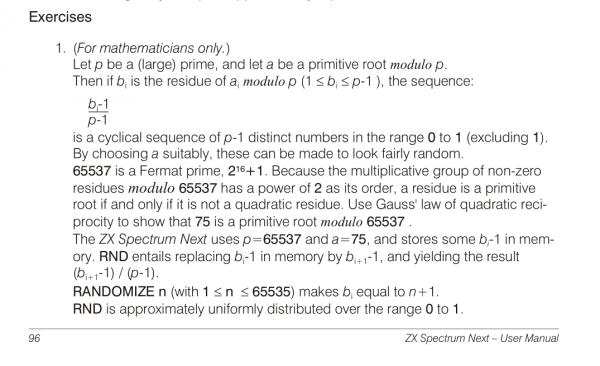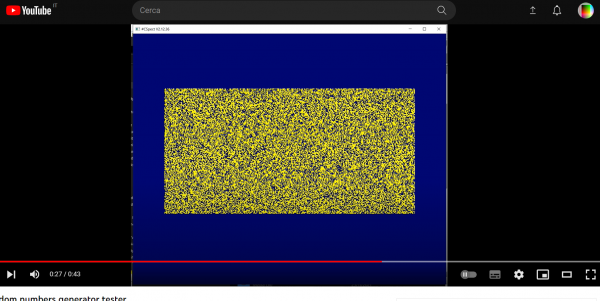# Programming the 80’s way #4

## Random Numbers in 16 bits

The ZX Spectrum Next’s manual explains in Chapter 11 how random numbers generator works.There is a useful disassembly (https://skoolkid.github.io/rom/dec/asm/9720.html) about the standard ROM routine, that using the “ROM calculator” in short uses the following formula

NEXT_SEED := ( ( 75 * ( SEED + 1 ) ) % 65537 ) – 1

where % is the “modulo operator”.

So starting with any SEED between 0 and 65535, the NEW_SEED is always between 0 and 65535, and the series that emerges is a fairly random sequence of integers that repeats itself after 65536 iterations.

Spectrum’s RND function simply returns  NEW_SEED divided by 65536.

Since a number larger than 65536 is involved (i.e. modulo base 65537), and Spectrum’s Forth usually provides only quotient/remainder routine that can divide a 32-bits integer giving a 16-bits integer result and a 16-bits remainder, for a long time I deemed impossible performing such an operation without “large divisor” quotient/remainder routine. And, this meant that it was impossible in my vForth too.

In this post, I show a clever achievement that circumvents the classic 16-bits limitation, in this case.

First of all, since Forth provides words that can handle 32-bits numbers addition and subtraction, I thought that I could “emulate” a quotient/remainder routine by “repeated subtractions”, and that was a fairly right path.

Immediately I saw that sutracting 65537 from a 32-bit integer, i.e. \$10001 in hexadecimal, was roughly equivalent to decrement both higher and lower 16-bits parts of it,
and that the reminder was simply some kind of “difference” between these two parts.

This was a good start, since instead of performing some huge division I just had to subtract two integers, and if the subtraction gave a negative number I just had to re-add 65537 to have it positive again.

Then I had to resolve these two limit conditions inherent using 16-bits integer Arithmetics :

1. when SEED is 65535, the first inner addition +1 produces a ZERO instead of a 65536 giving a wrong result.
2. 65537 is equivalent to 1.

So I exploited the following Forth final optimized definition that I listed below.

Here is a few seconds video (https://youtu.be/4Wh7r0ZHbSY) that shows how fast this routine is and how fast we can plot 65536 pixels using LAYER 1,2 graphics-mode.

decimal 23670 constant SEED
: next_seed ( n1 — n2 )
1+ ?dup
if
75 UM* 2dup U< if else – 1- then
else
-75 \ = 65461
then
;

needs graphics layer12

: tester
256 0 do
256 0 do
SEED @ next_seed SEED !
SEED @ 9 rshift 24 + SEED @ 511 and plot
loop
loop
;

cls tester_M.

This site uses Akismet to reduce spam. Learn how your comment data is processed.